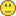New to QlikView

Discussion board where members can get started with QlikView.

Announcements
Highlighted
Esteemed Contributor

Offset in Class()

Hello Qlikers,

Can somebody help me to find out how Offset works in Class() Function??

=Class(10,5,'X',3)

How this 3 will be used in calculation while calculating expression?

Tags (5)
1 Solution

Accepted SolutionsMVP

Re: Offset in Class()

If no offset, the first range with Start with 0

If offset, the first range will end with that offset integer...

SIMPLE !

17 Replies
Esteemed Contributor III

Re: Offset in Class()

hi,

example

class( var,10,'x',5 ) with var = 23 returns '15<=x<25

Honored Contributor II

Re: Offset in Class()

Hi Balraj,

In class function 0 is normally the default starting point of the classification. By setting an offset you can change the default starting point.

Regards

KC

Best Regards,
KC
Esteemed Contributor

Re: Offset in Class()

Dear,

I already know this, I am asking how offset 5 is getting calculated here?MVP

Re: Offset in Class()

Seems to determine where you class starts from. Look at this example:

Table:

Class(Age, 5, 'X', 3) as Class1,

Class(Age, 5, 'X') as Class2;

LOAD Ceil(Rand()* 99) as Age

AutoGenerate 1000;

Output:Esteemed Contributor

Re: Offset in Class()

Jyothish,

Thats fine.  But I tried lot with Class() calculation, here are some example:

=Class(30,4,'X',9) gives 29<=X<33

=Class(30,4,'X',8) gives 28<=X<32

=Class(30,4,'X') gives 28<=X<32

How this offset values are being used here, like?

30-9+4   or 30+9-4 like this?

Esteemed Contributor

Re: Offset in Class()

Sunny, thanks for sharing this...My concern is how this offset value change the min and max value?MVP

Re: Offset in Class()

Change the min and max value? of the underlying field?

Esteemed Contributor

Re: Offset in Class()

mean to say,

For age 1, with offset how it is giving -2<=x<3MVP

Re: Offset in Class()

From Sunny's example

Class(Age, 5, 'X') as Class2

First Class starts from 0 to 5 as no offset is given... 1st class is 0<=X<5

But for below example

Class(Age, 5, 'X', 3) as Class1

Offset is 3..and Class range is 5... Here with offset the 1st Class with end with the offset value i.e. 3

So the range would be

SOMENUMBER <= X < 3

but you have Class Range is 5 so 3-5 = -2

Range is -2<=X<3